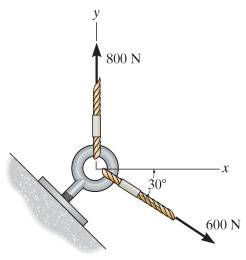# Resultant Force

Jud
I'm sure this problem is pretty trivial to most but apologies in advance, I'm just starting out sorry.

## Homework StatementI'm not sure how to go about working this out due to the 800N

## The Attempt at a Solution

F1 (800N) x-pos = 800 tan (90)?? , y-pos = ???
F2 (600N) x-pos = 600 cos(30) , y-pos = -600 sin(30)

## Answers and Replies

Gold Member
You can draw the parallelogram of forces, and the diagonal length (from your starting point) is the net force.

Or you can complete their conversion to vectors; F1 = 800 j, where j is the unit vector in the y-direction. Your F2 looks correct ... so now add them together!

Jud
You can draw the parallelogram of forces, and the diagonal length (from your starting point) is the net force.

Or you can complete their conversion to vectors; F1 = 800 j, where j is the unit vector in the y-direction. Your F2 looks correct ... so now add them together!

so,

F1 (800N) x-pos = 0 , y-pos = 800
F2 (600N) x-pos = 600 cos(30) , y-pos = -600 sin (30)

sqrt (519.615)^2 + (500)^2 = 721.1 N

is that correct?